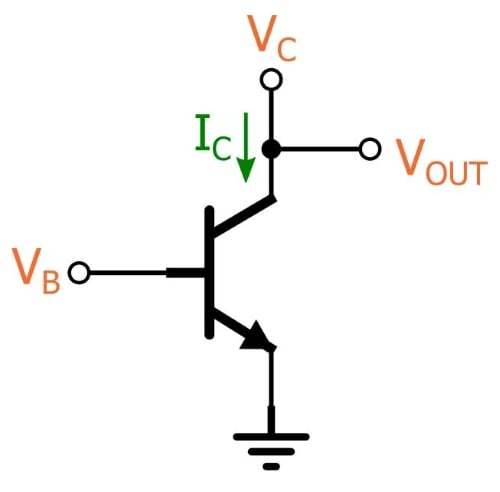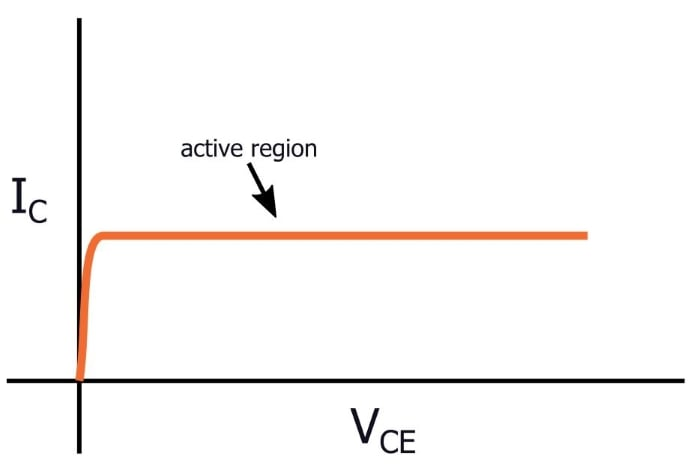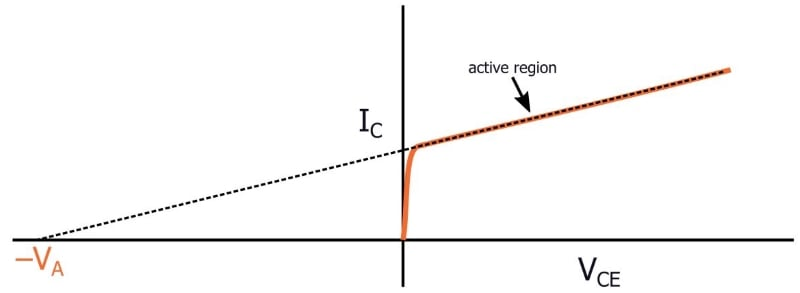Technical Article

# Understanding the Early Effect

April 02, 2018 by Robert Keim

## This technical brief discusses the Early effect and how it influences the amplification characteristics of a bipolar junction transistor.

This technical brief discusses the Early effect and how it influences the amplification characteristics of a bipolar junction transistor.

Related Information

In a previous article, I discussed channel-length modulation, which is something that occurs in a FET and causes the drain current to be dependent on the drain voltage (or more specifically the drain-to-source voltage). If we ignore channel-length modulation, the drain current in the saturation region is dependent only on the gate-to-source voltage and the physical characteristics of the FET. This would be pleasantly straightforward, but for some reason reality is often more complicated than we want it to be.

### The Early Effect

It turns out that an analogous phenomenon affects the operation of a bipolar junction transistor. A BJT doesn’t have a channel, though, so we need a different name; at some point people decided on the “Early effect,” after James Early, though you will see shortly that we could also call it “effective-base-width modulation.”

We usually say that the collector current of a BJT in active mode is equal to the base current multiplied by β. This implies that the gain of the transistor is not affected by the conditions at the output. This simplifies the analysis; also, a transistor operating in this way would have no distortion caused by a gain that changes as the output voltage changes.

This approach is acceptable for approximations and for the DC-bias portion of BJT design and analysis, but when we’re dealing with small-signal amplifiers, we need to consider the Early effect:

• First, let’s assume that the emitter voltage (VE) is constant. This means that we have two important voltages that are both referenced to an unchanging VE: the collector-to-emitter voltage (VCE) and the base-to-emitter voltage (VBE).
• Let’s also assume that the output is taken from the collector, such that variations in the output voltage (VOUT) are also variations of VCE.• If a small increase in VBE causes a large increase in VOUT, the collector-base junction becomes more reverse-biased (remember that in active mode the base-emitter junction is forward-biased and the collector-base junction is reverse-biased).
• This causes the junction’s depletion region to become wider, which in turn decreases the effective width of the base. We say “effective” because the physical width of the base region doesn’t change.
• The saturation current (IS) is inversely proportional to the effective width of the base, and thus an increase in VOUT will lead to an increase in IS.
• If IS increases, collector current also increases, as you can see from the following formula:

$$I_C=I_Se^{\frac{V_{BE}}{V_{T}}}$$

### Accounting for the Early Effect

If we hold VBE constant and plot collector current vs. VCE in active mode, the result for a simplified BJT would be a straight and flat line, indicating that the collector voltage has no effect on the gain, i.e., the relationship between VBE and IC. This is also what we observe in the above formula, which does not have a VCE term.If we incorporate the influence of effective-base-width modulation, the line is still straight but it is no longer flat. Rather, it has an upward slope, indicating that the output voltage affects the gain, because for a given VBE the collector current will be higher if the collector voltage is higher.If you trace this line back to the point at which it crosses the horizontal axis, you have the Early voltage, denoted by VA, and then you can more accurately determine collector current by using the following formula:

$$I_C=I_Se^{\frac{V_{BE}}{V_{T}}}\left(1+\frac{V_{CE}}{V_A}\right)$$

• Share• R
RICHARD A PHILLIPS April 03, 2018

I don’t quite understand several things in this article.  What is the significance of VE being constant?  If there was a resistor between the emitter and ground, VE would not be constant.  I don’t understand the equation for IC.  IS would be the saturation current which is where a further increase in VC does not increase IC at a given VB.  What does e^(VBE/VT) mean?  What is VT?  I can see how the effective width of the CB junction would increase if the voltage between the collector and base were to increase, but only intuitively.  I don’t think that I really understand the point of this.  I also don’t understand how projecting the active region to the horizontal axis represents the Early voltage.  In fact, I am not sure what the Early voltage is.  What is -VA?
I am not being critical.  I think that it is wonderful that you are writing about this.  I would just like to understand your article better.

Like.
•RK37 April 04, 2018
Hi Richard, These short articles are called "technical briefs," and they're intended for readers who are already familiar with most of the supporting information related to the topic at hand. I think I would need to write another article in order to answer your questions, and that's not really practical here in the comments :) You should be able to find some helpful information in the AAC textbook, in previous articles, or in existing forum threads. If you can't find all the answers that you're looking for, you could try posting a question in the forum.
Like.
• B
bmdaly August 04, 2019

Hi Robert,
Vout is always equal to Vc in the circuit drawn above. Is there a resistor missing?

Like.
•RK37 August 05, 2019
The output is taken directly from the collector, so yes, Vc is equal to Vout. I think that perhaps you are interpreting Vc as the supply voltage, but Vc simply refers to the voltage at the collector.
Like.
• B
bmdaly August 08, 2019
Thanks for the clarification - yes I was assuming Vc was Vcc.
Like.